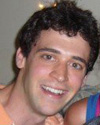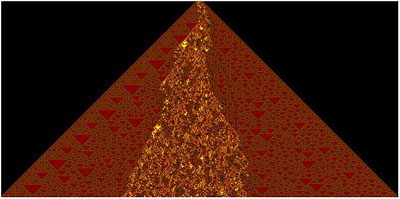#Wolfram Summer School

# Alumni# Stedman Wilson

Summer School

Class of 2007

## Bio

Stedman Wilson is a beginning PhD student in mathematics at UCLA. He received a Bachelor of Science degree from Stanford University with majors in mathematics and physics. His most memorable collegiate extracurricular activities include a year as a walk-on rower on the Stanford men’s crew team, two years in the community service group Side by Side, and two years as a pianist and vocalist for the band The Institute for the Advancement of Funk and Soul. Stedman has diverse interests in mathematics, and has considered pursuing analysis, combinatorics, logic, or number theory in graduate school. He also has a side interest in music theory, and (not surprisingly) the mathematical aspects of it. Stedman was exposed to A New Kind of Science during his freshman year, when Stephen Wolfram came to Stanford to speak about the book. He was first intrigued by NKS for what he felt was a much more intuitive approach to fundamental questions in physics. Later he became interested in the mathematics of computable functions (i.e. recursion theory), including NKS relevant topics such as computational universality. He is now also interested in the use of NKS methods to provide insight into mathematical problems, such as structure and randomness in number sequences. His favorite chapters of A New Kind of Science are Chapter 4 (Systems Based on Numbers), and Chapter 9 (Fundamental Physics).

## Project: The Distribution of Zeros of Periodic and Aperiodic Sinusoidal Sums

Consider the function F(x) = Σk sin(ai x), a sum of k sines. When k = 2, then the zeros of F are given by the families x = (2n𝜋)/(a1 + a2) and x = ((2n+1)𝜋)/(a1a2), where a1 and a2 are the frequencies of the two component sine waves. However, when k > 2, and some frequencies have irrational ratios, trigonometric sum and difference formulas cannot be used to factor and solve for the zeros algebraically. In this case, one method to obtain information about the distribution of the zeros of F is to discretize the real line into intervals of some natural length and ask how many zeros occur in each interval, forming a sequence of numbers which we call the Zero Sequence. The hope is that one can find some simple algorithm that will generate the Zero Sequence given as input the frequencies ai. In the k = 2 case this hope is realized, and the Zero Sequence can be generated using the spectrum representation of the numbers a1 and a2. The spectrum representation of a number is also related by generalized substitution systems to its continued fraction representation. However, in the k > 2 case, it seems that no relationship exists between the Zero Sequence and spectrum representations or generalized substitution systems. My primary tasks in this project were to search for some simple algorithm that can be used to produce the Zero Sequence of F when F is a sum of more than two sines and to determine whether the Zero Sequences correspond in a direct way to any digit expansion representations of the frequencies ai. Along the way I discovered a number of interesting properties of Zero Sequences, some related and some seemingly unrelated to the main goal of the project. However, in this project I suspect that most of the interesting questions about Zero Sequences of sinusoidal sums are intimately related to the question of whether Zero Sequences correspond to digit representations of the component frequencies.

## Favorite Outer Totalistic Three-Color Rule

Rule chosen: 11876355

My favorite 3-color outer totalistic cellular automaton is given by Rule 11876355. My favorite initial condition for this rule is the base 2 digits of 714690. I used the color scheme {0 -> Black, 1 -> Red, 2 -> Yellow}. When run with initial condition 1 this automaton is purely nested, and in fact emulates ECA Rule 90. However, when one increases the initial condition something much more complex happens. When run using inital condition 714690, using the above color scheme, this CA looks like a volcano with lava flow down the surface. The edges of the light cone of the CA produce exclusively red triangles, and are furthermore structured and periodic. However, in the center, there is a “lava flow” made up of yellow and black triangles in such a way that the lava almost glows. The shape of the lava flow appears completely random. Some 3-color CAs have “middle structures” which are complex yet symmetric around the central axis of the CA, but here there is no such symmetry in the contour of the lava flow. Also interesting is the fact that one can see the red background of the rest of the CA if one looks in between pieces of the “lava”. My favorite thing about this CA is that it looks like a volcano. From a purely CA point of view, what is most interesting is the fact that while all CAs have an overall symmetric triangle shape, this CA is slightly more flexible in allowing for an asymmetic middle structure. Take away the outer light cone and we have a simple algorithmic method for creating a very asymmetic contour.• 21st Annual Wolfram Summer School
• Bentley University, Waltham, MA, USA
• June 25–July 15, 2023